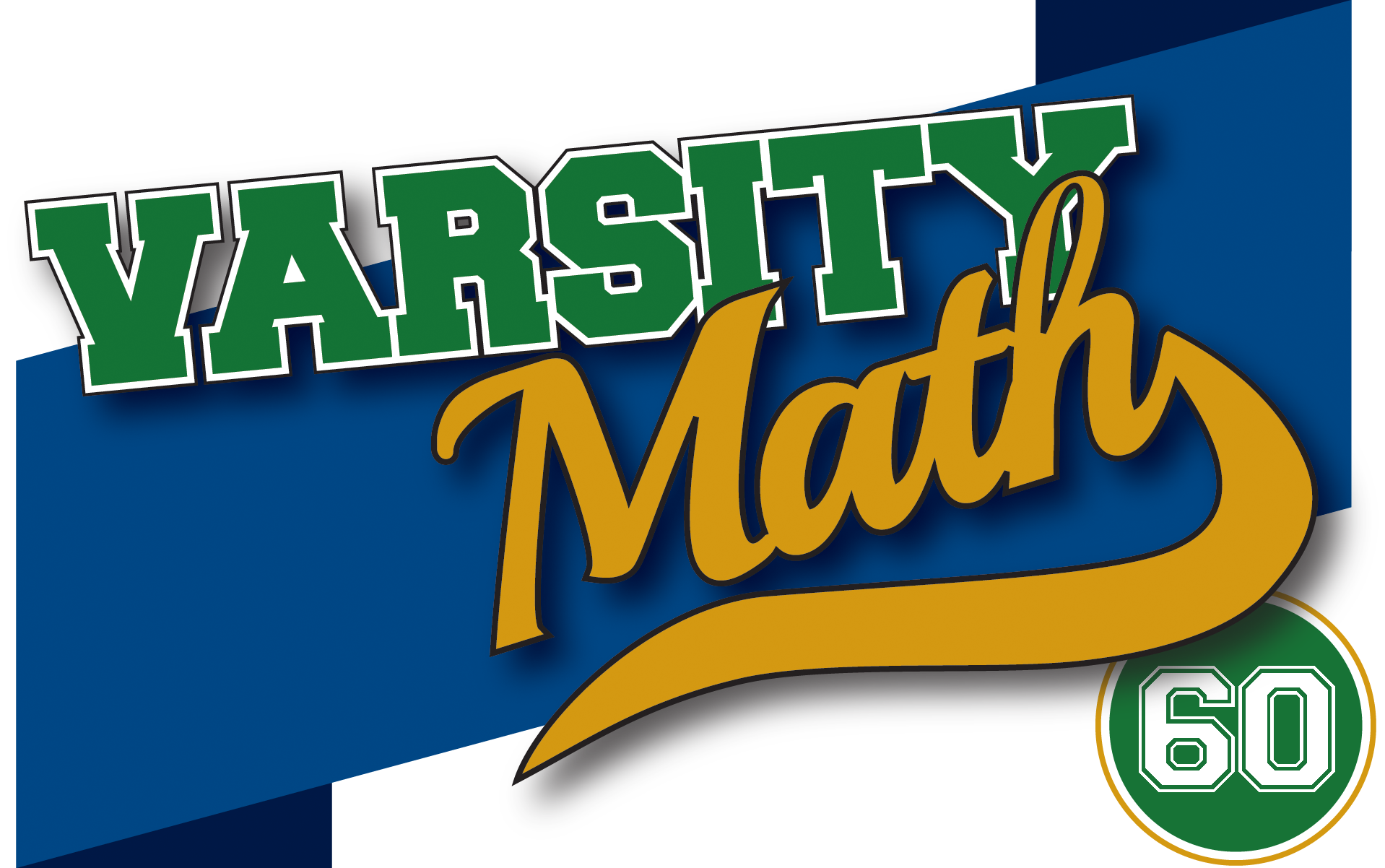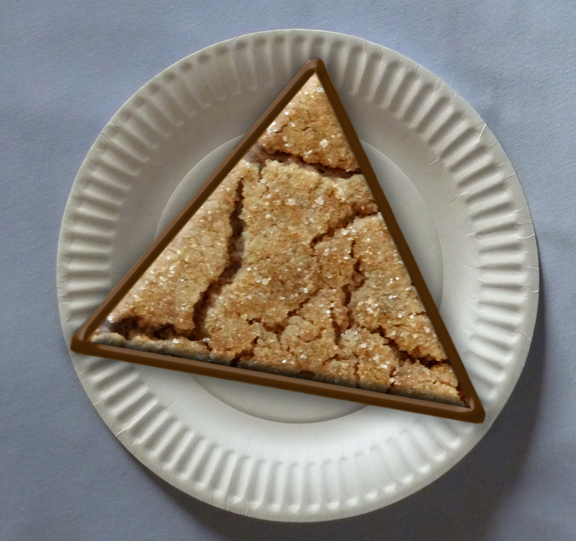## ________________

This week, the Varsity Math team is raising funds to cover its travel expenses to competitions.

## ________________

### Counting Cars

First, four members of the team try a car wash. They have ten cars to wash and only one hose, which is needed for rinsing but not for washing. Washing a car takes twelve minutes, but the labor can be split up any number of ways (and then the time required is divided by the number of people working together). Rinsing a car takes four minutes.

What is the minimum amount of time the four teammates will need to wash and rinse the ten cars?### Edible Division

The next day, the team tries a bake sale, including large equilateral triangle cookies outlined in chocolate. At the end of the sale, there is one cookie left, and three of the teammates are about to share it by cutting from the center to each of the corners, producing three identical pieces. Just then, a fourth teammate happens by.

How can the teammates divide the cookie so that each of the four of them gets the same amount of cookie and the same amount of chocolate?

## Solutions to week 59

Win-Win Move. Start by asking, “What’s the highest possible average any set of digits can have?” Obviously, nine — if the set consists of just the digit 9. But you can’t move one digit out of that set and increase the average (since then you would have the average of the empty set of digits, which is technically not defined). So what’s the second-highest possible average? Clearly, we can achieve that by taking the two highest digits: {8,9} has an average of 8.5, and there is no way to do better; all of the other digits are smaller than 8.5 and so will only drag down the average. Is there any way of including this as one of two sets containing each digit exactly once so that moving one digit will increase both averages? Yes! If you start with {1,2,3,4,5,6,7} and {8,9}, the averages are 4 and 8.5, and if you move the 8 from one set to the other, the averages are 4.5 and 9. So the highest possible average that can be increased by moving one digit is 8.5.

Square of Harmony. Let l and w be the length of Harmony’s original rectangle. You’re looking for the side of the square that has the same area as that rectangle. That area is lw, so the answer will be √lw, and in any case it is enough to know lw — then we can just take the square root. We’re told (l+w)/2 = 9 and 2/(1/l + 1/w) = 4. But look what happens if you simplify the left-hand side of that second equation. You get 4 = 2/((l+w)/lw) = 2lw/(l+w). So now the product of these is 9×4 = (l+w)/2 × 2lw/(l+w) = lw. So the side of Harmony’s square is √36 = 6, which is an integer as claimed.